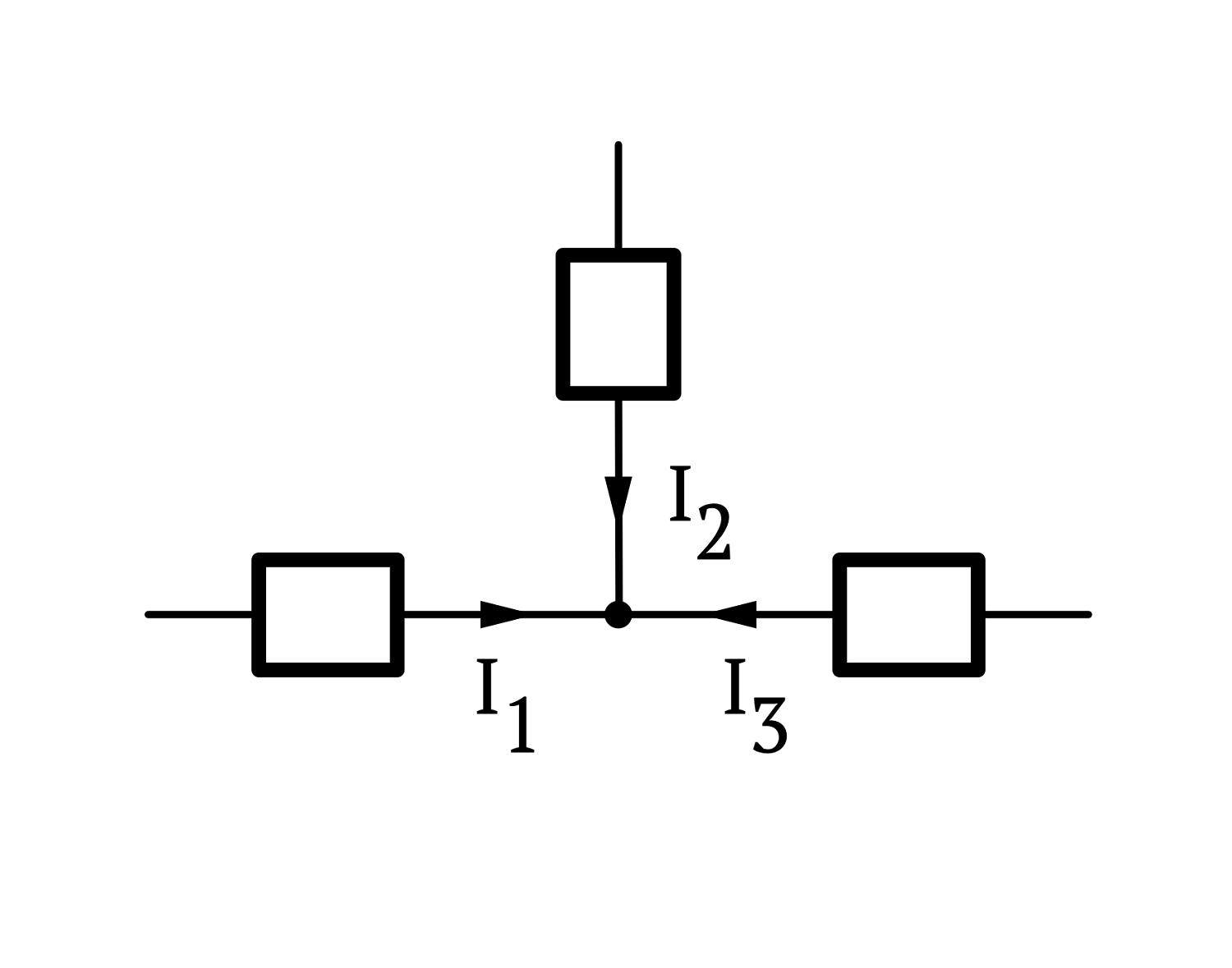# Kirchoff’s LawsWritten by

Circuit theory is a fancy name for an activity that we do all the time when we work with electronics. It refers to the calculation of voltages and currents in linear circuits. It is a formal branch of engineering that developed in the 19th century and has its own set of laws and theorems. The earliest of these—and one everyone is familiar with—is Ohm’s Law which describes the relationship between voltage and current in a circuit element. This was published in 1827.

Kirchoff’s Laws followed in 1845. Gustav Kirchoff was an interesting guy, developing theories in spectroscopy, radiation, and thermochemistry as well as in circuit theory. His laws seem sort of obvious, but they are the formal basis for things like calculating the values of resistors in series and parallel, as we shall see.

Figure 1 illustrates Kirchoff’s two laws. On the left, Kirchoff’s Current Law (KCL) simply states that the sum of all currents entering a node must equal zero. Note that the sign of the currents is important. At least one of the currents must be negative for this to make sense. In fact, KCL could equally be formulated as the sum of all currents leaving a node must equal zero. Just pick one convention or other and stick to it.FIGURE 1. Kirchoff’s Current Law (KCL) states that the sum of all currents entering (or leaving) a node in a circuit, must sum to zero. Similarly, Kirchoff’s Voltage Law (KVL) states that the sum of the voltages across the elements around any closed loop must be zero.

The corresponding law for voltages (KVL) is shown on the right-hand side of Figure 1. KVL states that the sum of the voltages across all of the elements around a closed loop must be zero. Again, sign is really important. You similarly need to pick a convention and stick to it. I have decided that the negative side of the element is first when traversing the loop clockwise, but I could equally have chosen it to be positive.

Note that a circuit “element” in this case can be any linear circuit element such as a resistor, capacitor, inductor, voltage source or current source etc.

Let’s look at a simple example of these laws in action. Consider the circuit in Figure 2. We want to determine the overall resistance Ro seen by the voltage source so we could calculate the current Io given Vo, for example.FIGURE 2. To reduce this resistor network to a single equivalent resistor we must first use KCL at the node marked A to find the Resistor R12 equivalent to the parallel combination. It is really important to watch the signs of the currents closely. It’s easy to make a mistake.

First, we need to reduce R1 and R2 to an equivalent single resistor R12. We start by looking at node A. KCL tells us that  Io  – I1I2  = 0 (noting the signs). This can be rearranged to Io =- I1 + I2. We can also see that the voltage across R1 and R2 and the notional equivalent resistor R12 are equal: V12 = V1 = V2. We can now divide the current equation by this voltage to get:

Or, using Ohm’s Law

Which is the formula for resistors in parallel that you will be familiar with. Now we can replace R1 and R2 with the new resistor R12 as shown in Figure 3.FIGURE 3. Now we can use KVL to determine the value of the R12 and R3. This is trivial, but that is only because Kirchoff’s laws that we can prove that the value of two resistors in series is the sum of their individual values.

Using KCL this time we can see that Vo – V12 – V3 = 0 (again watching our signs carefully) which can be written Vo = V12 + V3. Dividing this expression by the current that flows through all three gives:

or, by Ohm’s Law

You probably would have arrived at the same answer as this using a few short cuts, but the purpose of this exercise is to demonstrate that the rules you know for resistors in series and parallel, for example, are derived from Kirchoff’s Laws. They will also help you analyze more complex problems—especially in developing systems of equations to solve meshed circuits.

References:
“Gustav Kirchhoff.” In Wikipedia, April 18, 2021. https://en.wikipedia.org/w/index.php?title=Gustav_Kirchhoff&oldid=1018454580.
Khan Academy. “Circuit Analysis | Electrical Engineering | Science.” Accessed April 26, 2021. https://www.khanacademy.org/science/electrical-engineering/ee-circuit-analysis-topic.
Isaac Physics. “Isaac Physics.” Accessed April 26, 2021. https://isaacphysics.org/concepts/cp_kirchhoffs_laws.

 Keep up-to-date with our FREE Weekly Newsletter! Don't miss out on upcoming issues of Circuit Cellar. Subscribe to Circuit Cellar Magazine Note: We’ve made the May 2020 issue of Circuit Cellar available as a free sample issue. In it, you’ll find a rich variety of the kinds of articles and information that exemplify a typical issue of the current magazine.— ADVERTISMENT— —Advertise Here— Would you like to write for Circuit Cellar? We are always accepting articles/posts from the technical community. Get in touch with us and let's discuss your ideas.